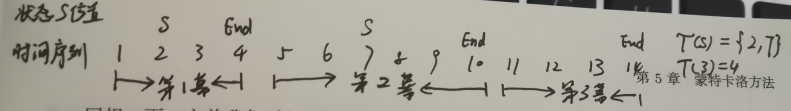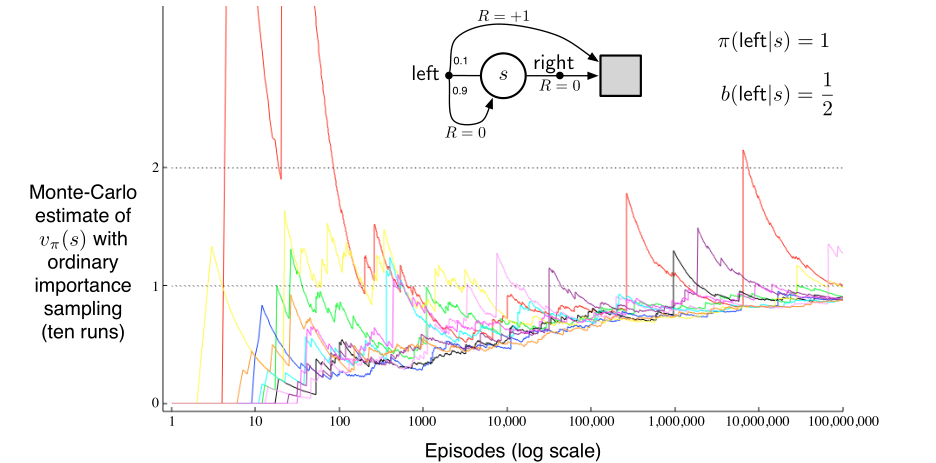# 强化学习（五）：蒙特卡洛采样方法

在强化学习（四）中，我们学习了如何使用动态规划法求解强化学习问题，我们还学习了策略评估和策略改进，以及广义策略迭代（GPI），事实上，动态规划能够很好地收敛到最优值，但是否动态规划就是最好的呢？显然不是。

v k + 1 ( s ) = ∑ a π ( a ∣ s ) ∑ s ′ , r p ( s ′ , r ∣ s , a ) [ r + γ v k ( s ′ ) ] v_{k+1}(s)=\sum_{a}\pi(a|s)\sum_{s',r}p(s',r|s,a)[r+\gamma v_{k}(s')]蒙特卡洛是一种随机采样策略，通常意义上说，给定一个确定的策略 π ( a ∣ s ) \pi(a|s) ，通过随机采样方式生成若干个采样序列，这些采样序列可以记做： S 0 , A 0 , R 1 , S 1 , A 1 , . . . , S T − 1 , A T − 1 , R T S_0,A_0,R_1,S_1,A_1,...,S_{T-1},A_{T-1},R_T 。我们知道对于一个强化学习的任务，其有两个设定，一个是分幕式设定，一个是折扣收益。本文所描述的蒙特卡洛也基于这两个设定。

#### 1、蒙特卡洛评估

延续GPI的思想。蒙特卡洛方法也是策略评估（预测）和策略控制（改进）之间的相互迭代。在策略评估阶段，首先给定一个初始化固定的策略 π \pi ，目标便是预测某状态的状态价值函数 v π ( s ) v_{\pi}(s) ，而对于某一个采样序列（幕），存在一些状态它们会被访问若干次，这在动态规划中是不会出现，但对于随机采样的序列是很大概率出现的，因此我们将蒙特卡洛方法其划分为两种：
（1）首次访问型（First-visit）:只统计每个状态首次被访问时的状态价值。
（2）每次访问型（Every-visit）：统计每个状态所有被访问后产生的状态价值。

如图所示，首次访问型的蒙特卡洛预测算法：蒙特卡洛评估过程中，每个采样序列之间是相互独立的，因此对每一个采样序列中的每个状态估计也是相互独立的，因此对于蒙特卡洛方法，回溯图是一条直线，其中空心点表示状态，实心点表示状态动作二元组：#### 2、蒙特卡洛控制

GPI的思想是策略评估与策略控制的交叉执行，在蒙特卡洛方法中也如此。
我们仔细思考一个问题，在获得一系类采样序列后，我们使用评估算法来尽可能的在当前策略 π \pi 下使得状态价值能够收敛，而在控制阶段，我们的目标则是选择一个最优动作。而蒙特卡洛方法因为其随机性，可能导致一个问题——有些状态可能一直没有被访问过，因此这里有三种解决策略：
（1）试探性出发：每一个状态动作二元组都有一定的概率被选作一个幕的起始点。这个假设能够直接保证每个状态动作都能被访问到，但应用场景很受限制；
（2） ϵ \epsilon 贪心和UCB：我们在强化学习（二）也讲过这一类方法，其在每次策略控制阶段，都设置一定概率进行随机选择。基于这一类的策略也被称为同轨策略；
（3）重要度采样：重要度采样时一种通过额外的行动策略分布来尽可能的试探未知的状态动作，来预测目标策略。下面将会分别对着三个蒙特卡洛控制进行讲解。

##### 2.1 基于试探性出发的蒙特卡洛控制

首先基于一个特定的策略 π \pi 生成一个采样序列，而所有的（状态-动作）二元组都有一定的概率被选做这个采样序列的初始部分，详细的算法如下图所示：试探性出发能够解决部分状态动作二元组无法访问的问题，但其也存在另一个问题：一般而言，很多应用领域是不适合随机初始状态的，因此不能够适合所有场景。

##### 2.2 基于软性策略的蒙特卡洛同轨策略控制

如果不使用基于试探性出发来实现，有没有别的方法呢？我们先介绍两个概念：

ϵ − \epsilon- 贪心指在策略控制阶段的某一个时刻状态 s t ， 有 s_{t}，有 ϵ \epsilon 的概率随机选择一个动作 a t a_t ，而有 1 − ϵ 1-\epsilon 的概率选择当前价值最高的动作 arg max ⁡ a Q ( s t , a ) \argmax_{a}Q(s_t,a) 。因此对于非价值最高的动作来说，都有 ϵ / ∣ A ∣ \epsilon/|A| 的概率被选中，而价值最高的动作则有 ϵ / ∣ A ∣ + 1 − ϵ \epsilon/|A| + 1-\epsilon 的概率被选中，因此每个动作被选中的概率均大于等于 ϵ / ∣ A ∣ \epsilon/|A| ，可以保证试探与开发之间的权衡。下图给出相关算法：##### 2.3 基于重要度采样的蒙特卡洛离轨策略控制

基于同轨的策略实际上是一种妥协，并不能学习到最优的动作，而基于离轨策略则可以学习到最终的最优策略。上面讲过，离轨策略是指用于评估或者改进的目标策略与具有试探性的行动策略不相同，换句话说，就是用行动策略 b b 去做试探性的采样，基于行动策略来改进目标策略 π \pi 。因此我们有如下的条件：
（1）目标策略 π \pi 下发生的动作都至少偶尔能在 b b 下发生。因为基于行动策略我们能够观察到的采样序列，但这个采样序列并不是我们学习和改进的目标策略生成的，因此我们必须保证目标策略能够发生的动作，行动策略也要能够发生，才能使得这个行动策略体现出试探性。形式化的描述即为 π ( a ∣ s ) > 0 \pi(a|s)>0 b ( a ∣ s ) > 0 b(a|s)>0
（2）重要度采样建立两种不同分布之间的关系。 重要度采样指在给定某个来自其他分布的条件下，估计某种分布的期望值的方法。例如给定行动策略 b ( a ∣ s ) b(a|s) ，这是一个满足 b b 策略的条件概率分布，我们希望用它来估计目标策略 π ( a ∣ s ) \pi(a|s) 的概率分布。重要度采样比是一种相对概率，其用来表示两种分布之间的相对关系。我们可以使用这个比值来将目标策略的期望 E x ∼ π [ v ( x ) ] \mathbb{E}_{x\sim\pi}[v(x)] 转移到行动策略的期望。公式如下：

E x ∼ π [ v ( x ) ] = ∑ x π ( x ) v ( x ) = ∑ x b ( x ) π ( x ) b ( x ) v ( x ) = E x ∼ b ( x ) [ π ( x ) b ( x ) v ( x ) ] \mathbb{E}_{x\sim\pi}[v(x)]=\sum_{x}\pi(x)v(x)=\sum_{x}b(x)\frac{\pi(x)}{b(x)}v(x)=\mathbb{E}_{x\sim b(x)}[\frac{\pi(x)}{b(x)}v(x)]

ρ t : T − 1 = ∏ k = t T − 1 π ( A k ∣ S k ) p ( S k + 1 ∣ S k , A k ) ∏ k = t T − 1 b ( A k ∣ S k ) p ( S k + 1 ∣ S k , A k ) = ∏ k = t T − 1 π ( A k ∣ S k ) b ( A k ∣ S k ) \rho_{t:T-1}=\frac{\prod_{k=t}^{T-1}\pi(A_k|S_k)p(S_{k+1}|S_k,A_k)}{\prod_{k=t}^{T-1}b(A_k|S_k)p(S_{k+1}|S_k,A_k)}=\prod_{k=t}^{T-1}\frac{\pi(A_k|S_k)}{b(A_k|S_k)}

（1） ρ t : T − 1 \rho_{t:T-1} ：表示从时刻 t t 开始一直到一幕的结束，当前状态的重要度采样比；
（2） T ( s ) T(s) ：状态s所处的幕序列的终止时间；
（3） T ( s ) \mathcal{T}(s) ：表示状态 s s 在每一幕中首次出现的位置的集合
（4） G t G_t ：表示遵循行动策略 b b 在当前状态 s t s_t 的回报；
（5） V ( s ) V(s) ：表示估计的目标策略 π \pi 在当前时刻 s t s_t 的价值。• 普通重要度采样（平均重要度采样）：
V ( s ) = ∑ t ∈ T ( s ) ρ t : T ( t ) − 1 G t ∣ T ( s ) ∣ V(s)=\frac{\sum_{t\in\mathcal{T}(s)}\rho_{t:T(t)-1}G_t}{|\mathcal{T}(s)|}
• 加权重要度采样：
V ( s ) = ∑ t ∈ T ( s ) ρ t : T ( t ) − 1 G t ∑ t ∈ T ( s ) ρ t : T ( t ) − 1 V(s)=\frac{\sum_{t\in\mathcal{T}(s)}\rho_{t:T(t)-1}G_t}{\sum_{t\in\mathcal{T}(s)}\rho_{t:T(t)-1}}
二者主要区别在于分母不同。#### 3、总结

蒙特卡洛方法很好的解决了动态规划无法事先知道状态转移等先验分布的问题，通过对采样序列的价值期望来预测价值也能保证收敛。因此蒙特卡洛有三大优势：
（1）符合实际的经历和应用场景；
（2）可以从模拟中进行学习；
（3）每一幕采样相互独立；

个人对MC收敛性有个简单的理解：如果说动态规划是一个非常理想化的内容，MC则是介于实际和理想化之间的一个过渡，上节课证明了DP的收敛性，可以推广，DP是一个对状态动作空间全部采样的一个特殊的MC，或者说是MC是DP的“子集”，如果MC能够执行几百万几千万次采样（绝大多数的MC例子都是很多次采样），结合贪心策略或重要度采样等方法，姑且可以认为可以达到DP的境界。但是，事实上，MC的收敛性并不是一个简单的MDP收敛问题，它还受到如何选择策略影响的，例如在有些时候，同样的算法，如果基于加权重要度采样可以收敛，但基于普通重要度采样就不会收敛，因此探讨MC收敛性不是那么简单的一件事情。
﻿
另外MC有个很强的假设——分幕式假设，这个在书的一开始就限定了，试想一下，像下棋可以认为是分幕式任务，每一幕是一局，每个采样序列就是落子，但是有的任务不满足这个设定，有些任务甚至是无限的（例如），所以MC在此就完全不可用；另外MC的一个弊端就是它必须遍历整个序列才能得到每个状态的价值期望，这很显然造成计算负担并不实用，，现如今一些强化学习系统也并不直接使用MC，就好比在关联规则数据挖掘里面，实际应用并不会去使用Apriori算法。

04-16222
03-07255506-128万+
09-223万+
07-303898
12-11312
04-08662
07-271万+
11-13900
04-2997